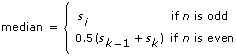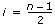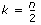Median Method

Measurement Studio 2012 for Visual Studio 2010 Help

Edition Date: August 2012

Part Number: 372636F-01

»View Product InfoDownload Help (Windows Only)

Calculates the median value of the input array by sorting the values of the input array and selecting the middle element(s) of the sorted array.

Namespace: NationalInstruments.Analysis.Math

Assembly: NationalInstruments.Analysis.Enterprise (in NationalInstruments.Analysis.Enterprise.dll) Version: 12.0.40.318

Syntax

Visual Basic (Declaration)
<SecurityPermissionAttribute(SecurityAction.Demand, Flags := SecurityPermissionFlag.UnmanagedCode)> _
Public Shared Function Median ( _
inputData As Double() _
) As Double

C#
[SecurityPermissionAttribute(SecurityAction.Demand, Flags = SecurityPermissionFlag.UnmanagedCode)]
public static double Median(
double[] inputData
)

Parameters

inputData
Type: System.Double []
The input array.

Return Value

The calculated median value of inputData.

Exceptions

ExceptionCondition
ArgumentException The number of samples in inputData is equal to zero.
ArgumentNullException inputData is null (Nothing in Visual Basic).
DllNotFoundException The analysis library cannot be found.
EntryPointNotFoundException A required operation in the analysis library cannot be found.
OutOfMemoryException There is not enough memory to carry out this operation.

Remarks

Let n represent the number of elements in inputData, and let s represent an array containing the values in inputData sorted in ascending order. The method finds the median using the following identity:where, and.

Examples

The following example calculates the median of the generated noise signal.
VB.NET
Dim inputData(1999) As Double
Dim median As Double
Dim gaussianNoise As GaussianNoiseSignal = New GaussianNoiseSignal(1.0, 17)

' Generate a Gaussian noise signal that represents inputData
inputData = gaussianNoise.Generate(1000.0, 2000)

median = Statistics.Median(inputData)
C#
double[] inputData = new double;
double median;
GaussianNoiseSignal gaussianNoise = new GaussianNoiseSignal(1.0, 17);

// Generate a Gaussian noise signal that represents inputData
inputData = gaussianNoise.Generate(1000.0, 2000);

median = Statistics.Median(inputData);